#### A Bayesian classifier

The probabilistic approach is most common in pattern classification. This results in a Bayesian classifier. Here it is assumed that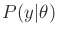and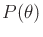are given. The distribution of features for a given class is indicated by. The overall frequency of class occurrences is given by. If large, preclassified data sets are available, then these distributions can be reliably learned. The feature space is often continuous, which results in a density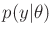, even thoughremains a discrete probability distribution. An optimal classifier,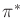, is designed according to (9.26). It performs classification by receiving a feature vector,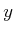, and then declaring that the class is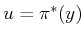. The expected cost using (9.32) is the probability of error.

Example 9..11 (Optical Character Recognition)   An example of classification is given by a simplified optical character recognition (OCR) problem. Suppose that a camera creates a digital image of a page of text. Segmentation is first performed to determine the location of each letter. Following this, the individual letters must be classified correctly. Let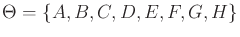, which would ordinarily include all of the letters of the alphabet.

Suppose that there are three different image processing algorithms:

1. [] Shape extractor: This returns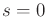if the letter is composed of straight edges only, and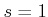if it contains at least one curve.
2. [] End counter: This returns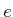, the number of segment ends. For example,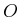has none andhas four.
3. [] Hole counter: This returns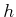, the number of holes enclosed by the character. For example,has none andhas one.
The feature vector is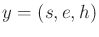. The values that should be reported under ideal conditions are shown in Figure 9.1. These indicate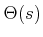,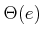, and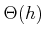. The intersection of these yields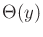for any combination of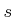,, and.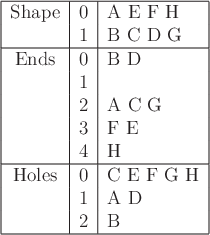Imagine doing classification under the nondeterministic model, with the assumption that the features always provide correct information. For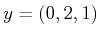, the only possible letter is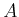. For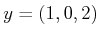, the only letter is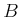. If each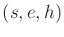is consistent with only one or no letters, then a perfect classifier can be constructed. Unfortunately,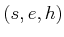is consistent with both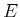and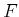. In the worst case, the cost of using (9.32) is.

One way to fix this is to introduce a new feature. Suppose that an image processing algorithm is used to detect corners. These are places at which two segments meet at a right (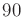degrees) angle. Let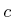denote the number of corners, and let the new feature vector be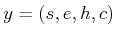. The new algorithm nicely distinguishesfrom, for which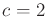and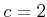, respectively. Now all letters can be correctly classified without errors.

Of course, in practice, the image processing algorithms occasionally make mistakes. A Bayesian classifier can be designed to maximize the probability of success. Assume conditional independence of the observations, which means that the classifier can be considered naive. Suppose that the four image processing algorithms are run over a training data set and the results are recorded. In each case, the correct classification is determined by hand to obtain probabilities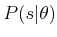,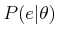,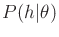, and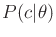. For example, suppose that the hole counter receives the letteras input. After running the algorithm over many occurrences ofin text, it may be determined that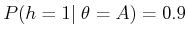, which is the correct answer. With smaller probabilities, perhaps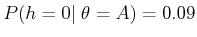and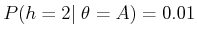. Assuming that the output of each image processing algorithm is independent given the input letter, a joint probability can be assigned as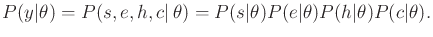(9.33)

The value of the priorcan be obtained by running the classifier over large amounts of hand-classified text and recording the relative numbers of occurrences of each letter. It is interesting to note that some context-specific information can be incorporated. If the text is known to be written in Spanish, thenshould be different than from text written in English. Tailoringto the type of text that will appear improves the performance of the resulting classifier.

The classifier makes its decisions by choosing the action that minimizes the probability of error. This error is proportional to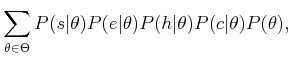(9.34)

by neglecting the constant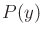in the denominator of Bayes' rule in (9.26).Steven M LaValle 2020-08-14